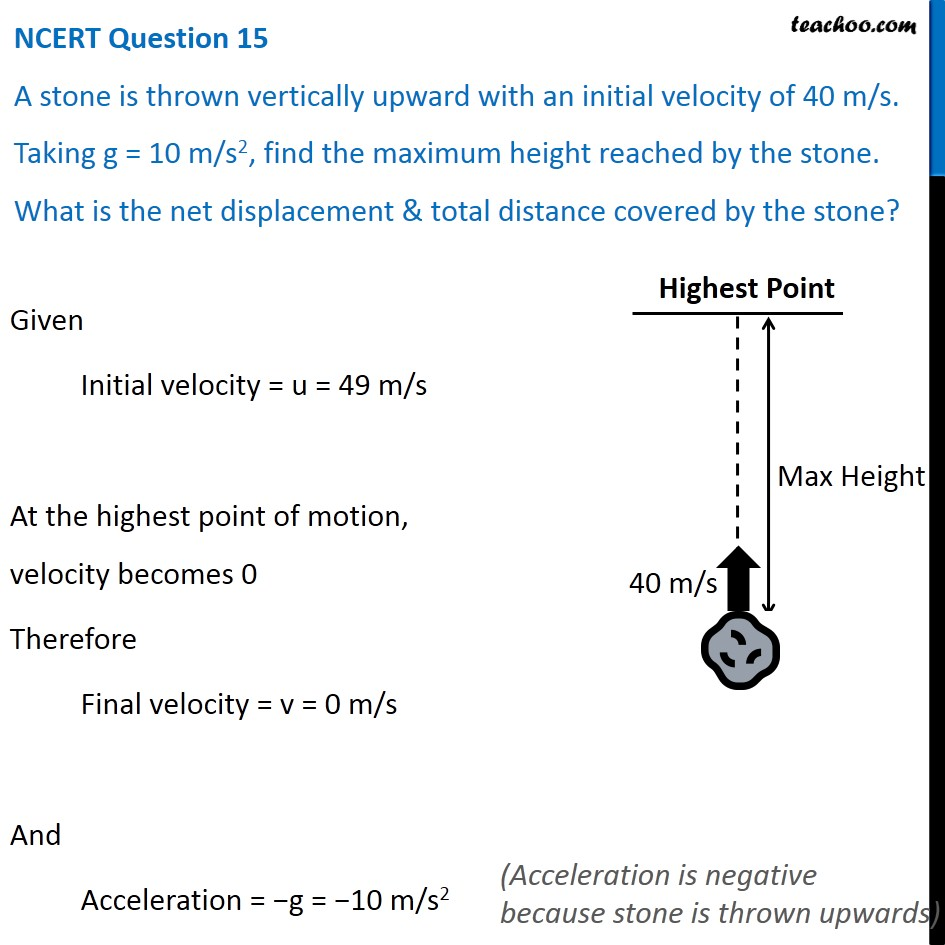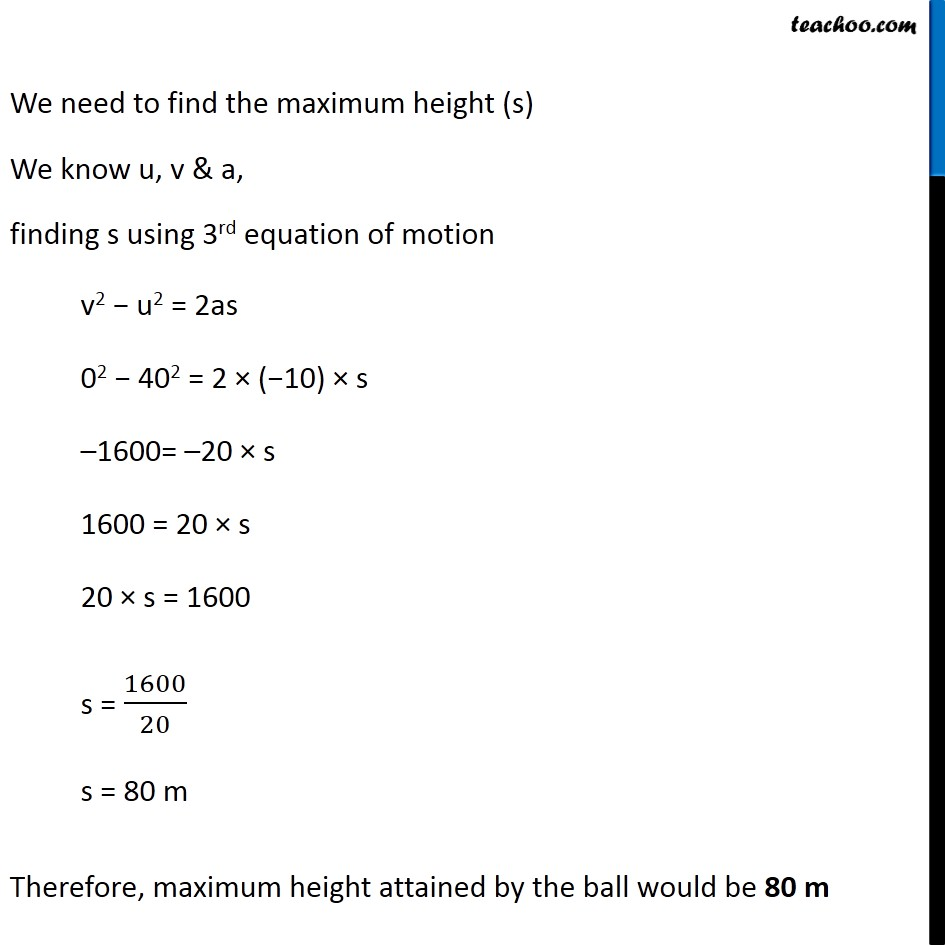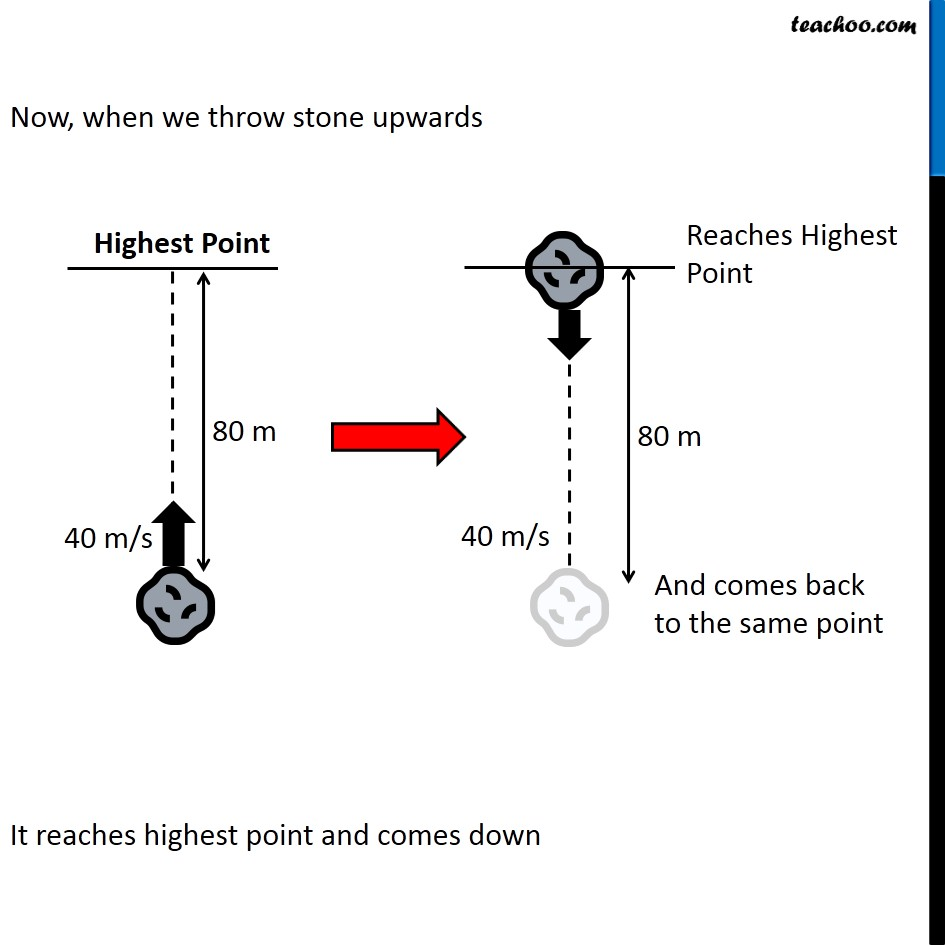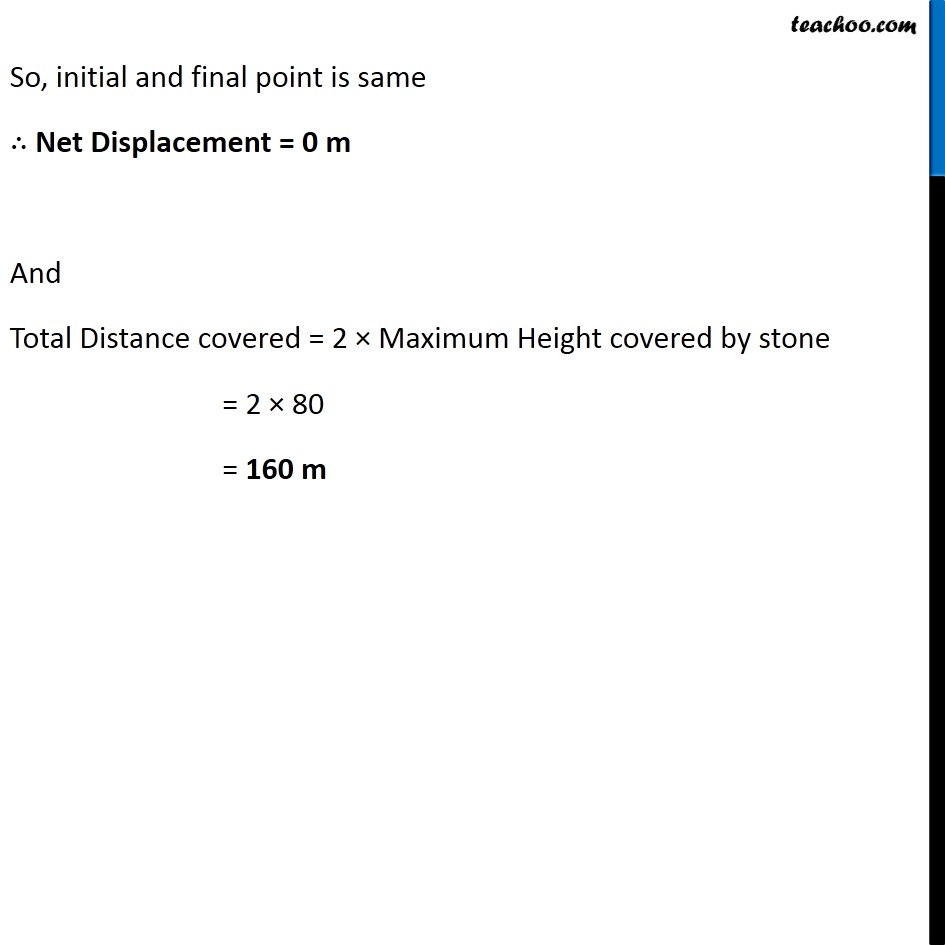NCERT Questions

Class 9
Chapter 10 Class 9 - GravitationLearn in your speed, with individual attention - Teachoo Maths 1-on-1 Class

### Transcript

NCERT Question 15 A stone is thrown vertically upward with an initial velocity of 40 m/s. Taking g = 10 m/s2, find the maximum height reached by the stone. What is the net displacement & total distance covered by the stone? Given Initial velocity = u = 49 m/s At the highest point of motion, velocity becomes 0 Therefore Final velocity = v = 0 m/s And Acceleration = −g = −10 m/s2 (Acceleration is negative because stone is thrown upwards) We need to find the maximum height (s) We know u, v & a, finding s using 3rd equation of motion v2 − u2 = 2as 02 − 402 = 2 × (−10) × s –1600= –20 × s 1600 = 20 × s 20 × s = 1600 s = 1600/20 s = 80 m Therefore, maximum height attained by the ball would be 80 m Now, when we throw stone upwards It reaches highest point and comes down Now, when we throw stone upwards It reaches highest point and comes down So, initial and final point is same ∴ Net Displacement = 0 m And Total Distance covered = 2 × Maximum Height covered by stone = 2 × 80 = 160 m